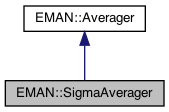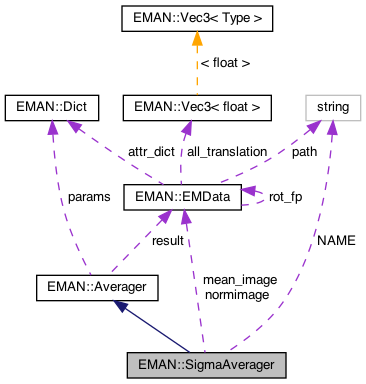EMAN2
EMAN::SigmaAverager Class Reference

SigmaAverager averages a list of images. More...

`#include <averager.h>`

Inheritance diagram for EMAN::SigmaAverager:[legend]
Collaboration diagram for EMAN::SigmaAverager:[legend]

## Public Member Functions

SigmaAverager ()

To add an image to the Averager. More...

EMDatafinish ()
Finish up the averaging and return the result. More...

string get_name () const
Get the Averager's name. More...

string get_desc () const

TypeDict get_param_types () const
Get Averager parameter information in a dictionary. More...

virtual void mult (const float &)
Multiply the result image by some floating point constant This is useful when weighting the input images prior to calling add_image - a situation where it is likely you want to divide by the sum of the weights. More...Public Member Functions inherited from EMAN::Averager
Averager ()

virtual ~Averager ()

virtual void add_image_list (const vector< EMData * > &images)
To add multiple images to the Averager. More...

virtual void set_params (const Dict &new_params)
Set the Averager parameters using a key/value dictionary. More...

## Static Public Member Functions

static AveragerNEW ()

## Static Public Attributes

static const string NAME = "sigma"

## Private Attributes

EMDatamean_image

EMDatanormimage

int ignore0

int nimg

int freenormProtected Attributes inherited from EMAN::Averager
Dict params

EMDataresult

## Detailed Description

SigmaAverager averages a list of images.

It optionally makes a sigma image.

Parameters
 sigma sigma value ignore0 if set, ignore zero value pixels

Definition at line 646 of file averager.h.

## ◆ SigmaAverager()

 SigmaAverager::SigmaAverager ( )

Definition at line 346 of file averager.cpp.

347 : mean_image(0), ignore0(0), normimage(0), freenorm(0), nimg(0)
348{
349
350}
EMData * normimage
Definition: averager.h:683
EMData * mean_image
Definition: averager.h:683

Referenced by NEW().

## Member Function Documentation

 void SigmaAverager::add_image ( EMData * image )
virtual

To add an image to the Averager.

This image will be averaged in this function.

Parameters
 image The image to be averaged.

Reimplemented from EMAN::Averager.

Definition at line 352 of file averager.cpp.

353{
354 if (!image) {
355 return;
356 }
357
358 if (nimg >= 1 && !EMUtil::is_same_size(image, mean_image)) {
359 LOGERR("%sAverager can only process same-size Image",
360 get_name().c_str());
361 return;
362 }
363
364 nimg++;
365
366 int nx = image->get_xsize();
367 int ny = image->get_ysize();
368 int nz = image->get_zsize();
369 size_t image_size = (size_t)nx * ny * nz;
370
371 if (nimg == 1) {
373 mean_image->set_size(nx, ny, nz);
374
376 result->set_size(nx, ny, nz);
377
378 ignore0 = params["ignore0"];
379 normimage = params.set_default("normimage", (EMData*)0);
380 if (ignore0 && normimage==0) { normimage=new EMData(nx,ny,nz); freenorm=1; }
381 if (normimage) normimage->to_zero();
382 }
383
384 float *mean_image_data = mean_image->get_data();
385 float *result_data = result->get_data();
386 float * image_data = image->get_data();
387
388 if (!ignore0) {
389 for (size_t j = 0; j < image_size; ++j) {
390 float f = image_data[j];
391 mean_image_data[j] += f;
392 if (result_data) {
393 result_data[j] += f * f;
394 }
395 }
396 }
397 else {
398 for (size_t j = 0; j < image_size; ++j) {
399 float f = image_data[j];
400 if (f) {
401 mean_image_data[j] += f;
402 if (result_data) {
403 result_data[j] += f * f;
404 }
405 normimage->set_value_at_fast(j,normimage->get_value_at(j)+1.0);
406 }
407 }
408 }
409}
EMData * result
Definition: averager.h:158
type set_default(const string &key, type val)
Default setting behavior This can be achieved using a template - d.woolford Jan 2008 (before there wa...
Definition: emobject.h:569
EMData stores an image's data and defines core image processing routines.
Definition: emdata.h:82
static bool is_same_size(const EMData *image1, const EMData *image2)
Check whether two EMData images are of the same size.
Definition: emutil.cpp:1224
string get_name() const
Get the Averager's name.
Definition: averager.h:654
#define LOGERR
Definition: log.h:51

## ◆ finish()

 EMData * SigmaAverager::finish ( )
virtual

Finish up the averaging and return the result.

Returns
The averaged image.

Implements EMAN::Averager.

Definition at line 411 of file averager.cpp.

412{
413 if (mean_image && nimg > 1) {
414 size_t image_size = (size_t)mean_image->get_xsize() * mean_image->get_ysize() * mean_image->get_zsize();
415 float * mean_image_data = mean_image->get_data();
416 if (!ignore0) {
417 for (size_t j = 0; j < image_size; ++j) {
418 mean_image_data[j] /= nimg;
419 }
420
421 float * result_data = result->get_data();
422
423 for (size_t j = 0; j < image_size; ++j) {
424 float f1 = result_data[j] / nimg;
425 float f2 = mean_image_data[j];
426 result_data[j] = sqrt(f1 - f2 * f2);
427 }
428
429 result->update();
430 }
431 else {
432 for (size_t j = 0; j < image_size; ++j) {
433 if (normimage->get_value_at(j)>0) mean_image_data[j] /= normimage->get_value_at(j);
434 }
435
436 float * result_data = result->get_data();
437
438 for (size_t j = 0; j < image_size; ++j) {
439 float f1 = 0;
440 if (normimage->get_value_at(j)>0) f1=result_data[j] / normimage->get_value_at(j);
441 float f2 = mean_image_data[j];
442 result_data[j] = sqrt(f1 - f2 * f2);
443
444 result->update();
445 }
446 }
447
448 mean_image->update();
449 mean_image->set_attr("ptcl_repr",nimg);
450
451 result->set_attr("ptcl_repr",nimg);
452
453 if (freenorm) { delete normimage; normimage=(EMData*)0; }
454
455 return result;
456 }
457 else {
458 LOGERR("%sAverager requires >=2 images", get_name().c_str());
459 }
460}
EMData * sqrt() const
return square root of current image

References freenorm, get_name(), ignore0, LOGERR, mean_image, nimg, normimage, EMAN::Averager::result, and sqrt().

## ◆ get_desc()

 string EMAN::SigmaAverager::get_desc ( ) const
inlinevirtual

Implements EMAN::Averager.

Definition at line 659 of file averager.h.

660 {
661 return "Computes the standard deviation of images";
662 }

## ◆ get_name()

 string EMAN::SigmaAverager::get_name ( ) const
inlinevirtual

Get the Averager's name.

Each Averager is identified by a unique name.

Returns
The Averager's name.

Implements EMAN::Averager.

Definition at line 654 of file averager.h.

655 {
656 return NAME;
657 }
static const string NAME
Definition: averager.h:680

References NAME.

## ◆ get_param_types()

 TypeDict EMAN::SigmaAverager::get_param_types ( ) const
inlinevirtual

Get Averager parameter information in a dictionary.

Each parameter has one record in the dictionary. Each record contains its name, data-type, and description.

Returns
A dictionary containing the parameter info.

Reimplemented from EMAN::Averager.

Definition at line 669 of file averager.h.

670 {
671 TypeDict d;
672 //d.put("sigma", EMObject::EMDATA, "sigma value");
673 d.put("normimage", EMObject::EMDATA, "In conjunction with ignore0, the number of non zero values for each pixel will be stored in this image.");
674 d.put("ignore0", EMObject::INT, "if set, ignore zero value pixels");
675 return d;
676 }

References EMAN::EMObject::EMDATA, EMAN::EMObject::INT, and EMAN::TypeDict::put().

## ◆ mult()

 virtual void EMAN::SigmaAverager::mult ( const float & s )
inlinevirtual

Multiply the result image by some floating point constant This is useful when weighting the input images prior to calling add_image - a situation where it is likely you want to divide by the sum of the weights.

Hence call mult after all of the weighted images have been added.

Parameters
 s the scaling factor.
Exceptions
 NullPointerException if the EMData pointer (result) is NULL

Reimplemented from EMAN::Averager.

Definition at line 678 of file averager.h.

678{ }

## ◆ NEW()

 static Averager * EMAN::SigmaAverager::NEW ( )
inlinestatic

Definition at line 664 of file averager.h.

665 {
666 return new SigmaAverager();
667 }

References SigmaAverager().

## ◆ freenorm

 int EMAN::SigmaAverager::freenorm
private

Definition at line 686 of file averager.h.

## ◆ ignore0

 int EMAN::SigmaAverager::ignore0
private

Definition at line 684 of file averager.h.

## ◆ mean_image

 EMData* EMAN::SigmaAverager::mean_image
private

Definition at line 683 of file averager.h.

## ◆ NAME

 const string SigmaAverager::NAME = "sigma"
static

Definition at line 680 of file averager.h.

Referenced by get_name().

## ◆ nimg

 int EMAN::SigmaAverager::nimg
private

Definition at line 685 of file averager.h.